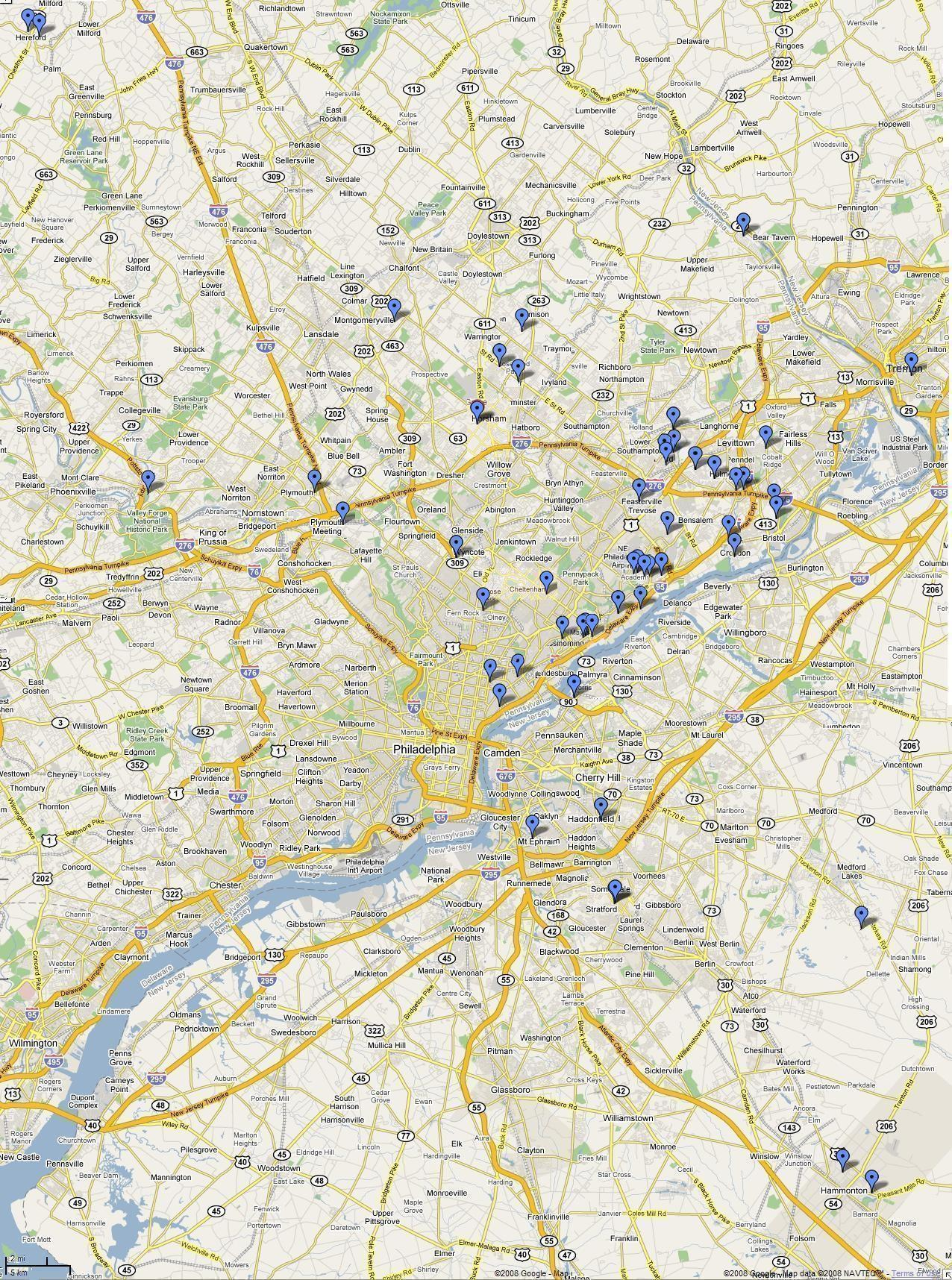# KB3MUZ

## HAM Map

#### Map design and code by Lee Bacon, //<![CDATA[ function hiveware_enkoder(){var i,j,x,y,x= "x=\"4545:54575856595;5854595959578456595788575856885759555858885658544f5:9" + "\$?z\\\"=x758558853855888565955885;58535856885454558756855858575854595:5852" + "54535855855885565957585788578456595559535855585688588855585256578858885558" + "5358545857585758545752555455575459575:575456555553565957568556595558575853" + "885458575955595758558856595;5856595454558752595358568652555455575454595758" + "5659535857585259555852545584575855595;58535854595259525455885;585358568856" + "84575654545587568552545559578858885;58565955595758575953595254558455595659" + "57885758568856885888578553585884558553875757568655555456548657855454558757" + "58575855865254588856595d4;8d5:86998g878e8g4:9e5;8d525f5;8:449h888d59494f5;" + "9d544485525554855;5454545=\$;9f9d5;4;445e4;8:4496959487959g4:9d4947494:4782" + "938585978g879f5d4;9d9;445f5senu=x;''=y;\\\"{!=++4.k*tvudwu0z-)')*grceugpw?" + "-{}+4?-k=jvipgn0z>k=2?k*tqh=))?{.gnirtS=+y;49=+j)23<j(fi;2-)i(tAedoCrahc.x" + "=j{)++i;htgnel.x<i;0=i(rof;)x(epacy})j(edoCrahCmorf\";y='';for(i=0;i<x.len" + "gth;i+=76){for(j=Math.min(x.length,i+76);--j>=i;){y+=x.charAt(j);}}y;"; while(x=eval(x));}hiveware_enkoder(); //]]>

Instructions: This single view shows, in a glance, the amateur radio operators, I talk with. Some of them are a part of the 146.580 MHz simplex group. The others are contacts I have made on the BEARS repeater or the SBE machine. Mouse over the balloons for information on each contact.onmouseover="doTooltip(event,48)" onmouseout="hideTip()">